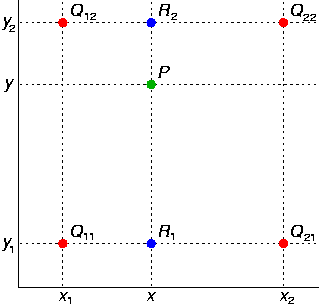## Coding Bilinear Interpolation

Pages: 1 2

When performing image transformation and manipulation techniques, it is often necessary to employ some sort of interpolation or filtering in order to obtain a good image quality. For example, if you scale an image, you can determine the final color of each pixel by either some basic nearest neighbor method, or a more advanced interpolation method. However, an interpolation method will invariably offer better final image quality. In this tutorial, we’ll be writing a function to rotate an image, using bilinear interpolation. This tutorial also demonstrates how to perform a high quality image rotate transformation, however, that is not the focus of this tutorial, but rather the example transform being performed.

## Why interpolation is used after image transforms

Image transforms like scaling, rotating, twisting or otherwise warping, effectively move pixels around in the image. The result is that the pixels in the final image do not directly map to a single original pixel in the original image. Suppose after rotation, a pixel should have the same color as pixel (3.4, 5.6) in the original image. The only problem is that there is no such thing as fractional pixels! Instead of simply choosing the color of the nearest available pixel, (3,6), we can get better results by intelligently averaging the colors of the four closest pixels. In this case, the values of pixels (3,5) (3,6) (4,5) and (4,6) would be intelligently averaged together to estimate the color of an imaginary pixel at (3.4, 5.6). This is called interpolation, and it is used after image transforms to provide a smooth, accurate and visually appealing images.

## Understanding bilinear interpolationWe can best understand bilinear interpolation by looking at the graphic here. The green P dot represents the point where we want to estimate the color. The four red Q dots represent the nearest pixels from the original image. The color of these four Q pixels is known. In this example, P lies closest to Q12, so it is only appropriate that the color of Q12 contributes more to the final color of P than the 3 other Q pixels.

## Calculating bilinear interpolation

There are several ways equivalent ways to calculate the value of P. An easy way to calculate the value of P would be to first calculate the value of the two blue dots, R2, and R1. R2 is effectively a weighted average of Q12 and Q22, while R1 is a weighted average of Q11 and Q21.

R1 = ((x2 – x)/(x2 – x1))*Q11 + ((x – x1)/(x2 – x1))*Q21

R2 = ((x2 – x)/(x2 – x1))*Q12 + ((x – x1)/(x2 – x1))*Q22

After the two R values are calculated, the value of P can finally be calculated by a weighted average of R1 and R2.

P = ((y2 – y)/(y2 – y1))*R1 + ((y – y1)/(y2 – y1))*R2

The calculation will have to be repeated for the red, green, blue, and optionally the alpha component of.

## Quality comparison

Below are two images of a turtle rotated by the code later on in this article. You’ll need to examine the full size images to tell the differences. One image uses bilinear interpolation while the other simply chooses pixel the nearest upper left pixel to determine the color o each pixel after the rotation. Notice how the image with bilinear interpolation is much smoother, especially in the pattern on the front arm. The image which uses a more basic, non-interpolation approach is clearly inferior in terms of image quality.

The source code for this tutorial is available on the next page, and may be used for any purpose.

Pages: 1 2